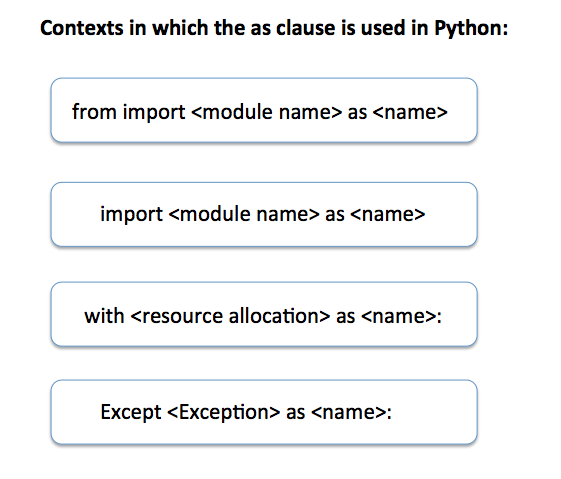# As Keyword In Python

## Overview:

The as clause in python is used in the following contexts:

• Accompanied by the with statement to name the resource being allocated

• In the from…import statement and the import statement as a means to bind the module to a name

• In the except statement to associate a name to the exception being passedFig-1: The as keyword in Python

## Example 1 - as clause used in import statement:

 # as clause used to bind the name np for the numpy module import numpy as np   radians = np.pi   # calculate sine, cosine and tan values for np.pi radians sineValue       = np.sin(radians) cosineValue     = np.cos(radians) tanValue        = np.tan(radians)   # print the sine, cosine and tan values print("Sine of %f radians:%f"%(radians, sineValue)); print("Cosine of %f radians:%f"%(radians, cosineValue)); print("Tangent of %f radians:%f"%(radians, tanValue));

## Output 1:

 Sine of 3.141593 radians:0.000000 Cosine of 3.141593 radians:-1.000000 Tangent of 3.141593 radians:-0.000000

## Example 2 - as clause used in from import statement:

 # as clause used to bind the name plot, with pyplot module from matplotlib import numpy as np from matplotlib import pyplot as plot   sampleSequence      = np.arange(0,400,10) magnitudeSequence   = np.sin(2*np.pi*sampleSequence*1/400)   plot.plot(sampleSequence,magnitudeSequence) plot.title('Sinusoid') plot.xlabel('Sample') plot.ylabel('Amplitude')   plot.show()

## Output 2:

 with open('sample.txt') as fileObject:     fileContents = fileObject.read()   print("Text file contents:") print(fileContents)

## Example 3 - as clause accompanied by the with statement:

 with open('sample.txt') as fileObject:     fileContents = fileObject.read()   print("Text file contents:") print(fileContents)

## Output 3:

 Text file contents: sample text

## Example 4 – along with except clause:

 try:     fileContents    = ""     with open('sample.txt') as fileObject:         fileContents = fileObject.read()         print("Text file contents:")         print(fileContents)    except FileNotFoundError as ex:     print(ex)

## Output 4:

 [Errno 2] No such file or directory: 'sample.txt'

Copyright 2019 © pythontic.com